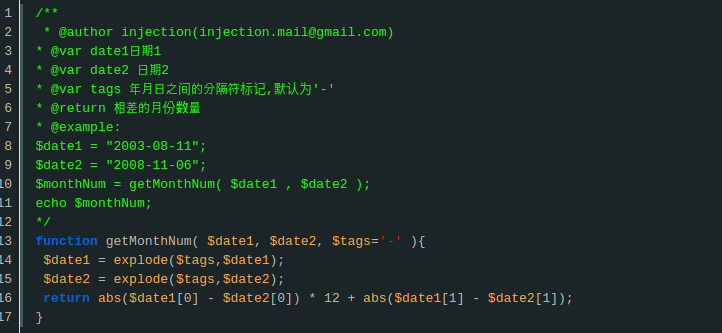# php 获取两个日期之间相隔的年、月、日、时、分、秒

在PHP中如果我们想获取两个时间日期之间相隔有多少天，多少个月，你会怎么做呢？我们先看看网友提供的一些参考：以上图片仅作为列子，无意冒犯，如有侵权请联系删除

<?php
/**
* @param $startDateTime 开始时间 * @param$endDateTime 结束时间
* @return array
*/
function timeDiff($startDateTime,$endDateTime) {
$startDateTime = new DateTime($startDateTime);
$endDateTime = new DateTime($endDateTime);
$interval =$startDateTime->diff($endDateTime);$formatMap = [
'y' => 'year',
'm' => 'month',
'd' => 'day',
'h' => 'hour',
'i' => 'minute',
's' => 'second',
'days' => 'days',
];
$returnData = []; foreach ($formatMap as $key =>$val) {
$returnData[$val] = $interval->{$key};
}
return $returnData; } ?> <?php ...$res = timeDiff('2018-02-11', ''2018-03-01');

print_r($res); // 已下为结果： /** * Array * ( * [year] => 0 // 相差的 年 数 * [month] => 0 // 相差的 月 数 * [day] => 18 // 相差的 天 数 * [hour] => 0 // 相差的 小时 数 * [minute] => 0 // 相差的 分钟 数 * [second] => 0 // 相差的 秒 数 * [days] => 18 // 相差总的天数 * ) **/ // 计算总的月份$res['year'] * 12 + \$res['month'];